# Volume

## Introduction to Volume

When you walk into McDonald’s and order Coca Cola, you get to choose between three variants, regular, medium and large. What is it that’s different between these three choices? Well, one look at the glasses that are used to serve them tells you that the only thing different is the amount of coca cola contained within!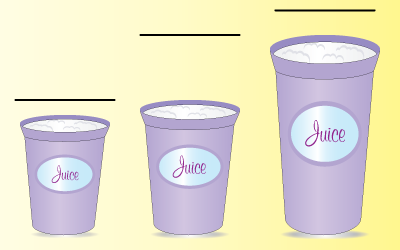## What is Volume?

The best way to visualize volume is to think of it in terms of the space enclosed/occupied by any 3-dimensional object or solid shape.

Do this simple exercise at home:

• Take a rectangular sheet of paper of length l cm and width h cm.

• Join the opposite sides of the sheet of paper without folding/creasing the sheet.

• See! You have made a 3-D object that encloses space within, from a 2-D sheet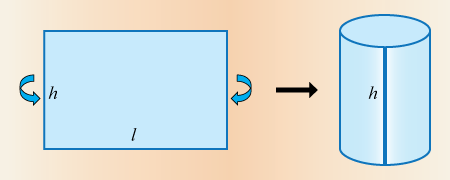## How is it important?

### Units of Volume

Given that volume has 3 dimensions, it has a cubic measure of length.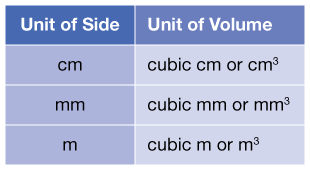However, while the standard unit of measurement internationally is cubic metre or cubic centimetre, colloquially the most used term is litres or millilitres.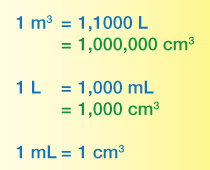Now that we are familiar with the units, let’s take a look at how to calculate the volume for a few common shapes.

### Volume of a cuboid

A prism with different values for length, breadth and height, the volume enclosed can be calculated as follows.

Let us represent the length as ‘$${l}$$’, breadth as ‘$${b}$$’ and height as ‘$${h}$$’. Then, the \begin{align}{\text{Volume of prism}} = l \times b \times h = lbh\end{align}

The way to try and visualise this is to think of it like the area of a rectangle multiplied by a third, new dimension, the height.

Have a look at the image below to see how the volume of a rectangular prism (or cuboid) can be calculated.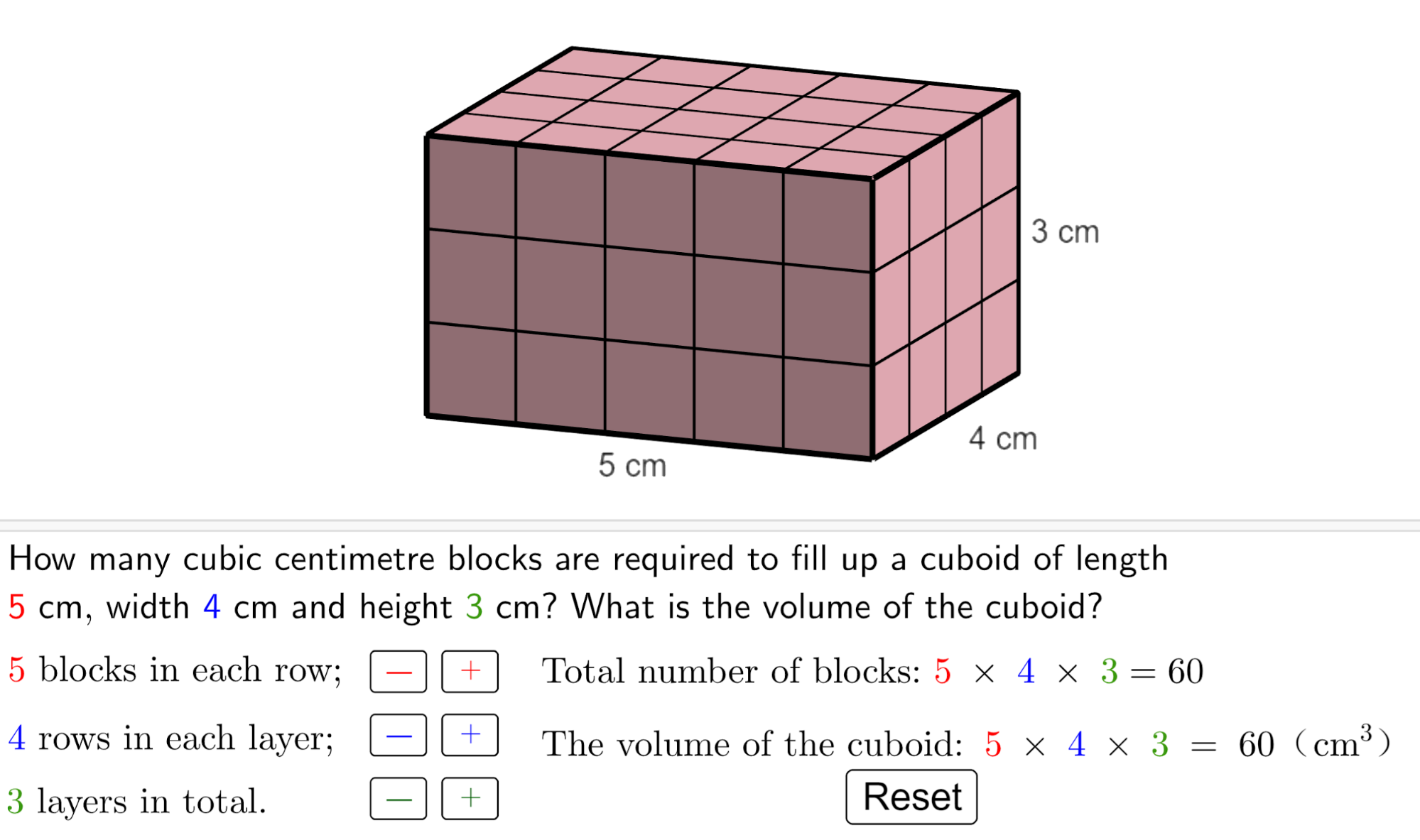### Volume of cube

A cube is a special case of the cuboid where all three sides are equal in measure. If we represent this equal value as ‘$${a}$$’, then the cube is said to have side ‘$${a}$$’. The volume of this cube can then be calculated as:

\begin{align}{\text{Volume of cube}} = a \times a \times a = {a^3}\end{align}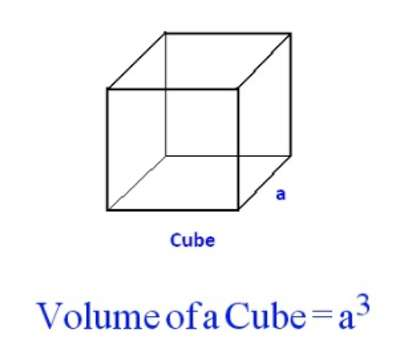### Volume of cylinder

A cylinder is a tube-like structure with circular faces of the same radius at either end joined by the planar circular surface.

Think of it as the area of a circle multiplied by a third new dimension, the height.

If you consider $${r}$$ as the radius of the circular base (and top) and $${h}$$ as the height of the cylinder, then the volume of the cylinder is expressed as follows:

\begin{align}{\text{Volume of cylinder}} = \pi \times r \times r \times h= {\pi r^2 h}\end{align}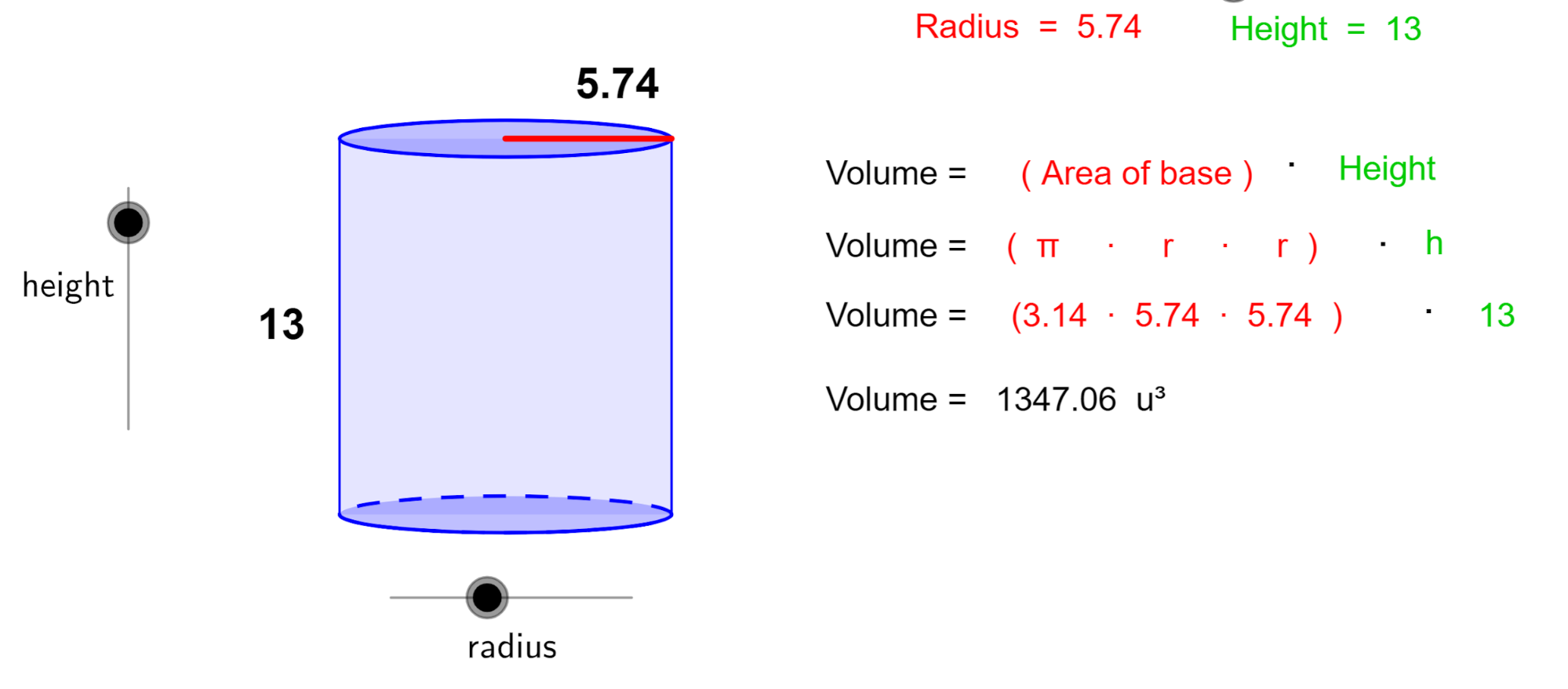### Volume of pyramid

A pyramid is a shape formed by a base, usually a square or a triangle, although pyramids with bases with greater than 4 are also possible and planar triangular surfaces.

\begin{align}\text{Volume of a pyramid} &= \frac{1}{3} \times \text{area of base} \times \text{height}\\& = \frac{1}{3} \times {a^2} \times h\text{ }\\&\left( \text{here }h\text{ is the height of the pyramid, and }\\ a\text{ is the area of the base} \right)\end{align}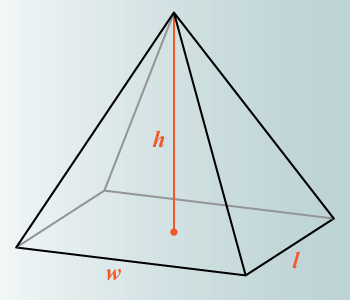### Volume of cone

The difference between a cone and a pyramid is that the base of a cone is circular whereas the base of a pyramid is a square. Also, the cone has a curved surface instead of a planar surface.

Think of the cone you get when you order an ice cream.

\begin{align}{\text{Volume of a cone}} = \frac{1}{3} \times \pi \times r \times r \times h = \frac{1}{3} \times \pi \times {r^2} \times h\end{align}

\begin{align}\left( \text{here }h\text{ is the height of the cone, } r\text{ is the radius of }\\ \text{the circular base, and } \pi \text{ is a constant} \right)\end{align}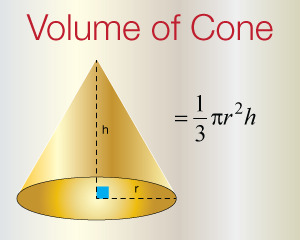## Important Topics

More Important Topics
Numbers
Algebra
Geometry
Measurement
Money
Data
Trigonometry
Calculus
More Important Topics
Numbers
Algebra
Geometry
Measurement
Money
Data
Trigonometry
Calculus
Learn from the best math teachers and top your exams

• Live one on one classroom and doubt clearing
• Practice worksheets in and after class for conceptual clarity
• Personalized curriculum to keep up with school